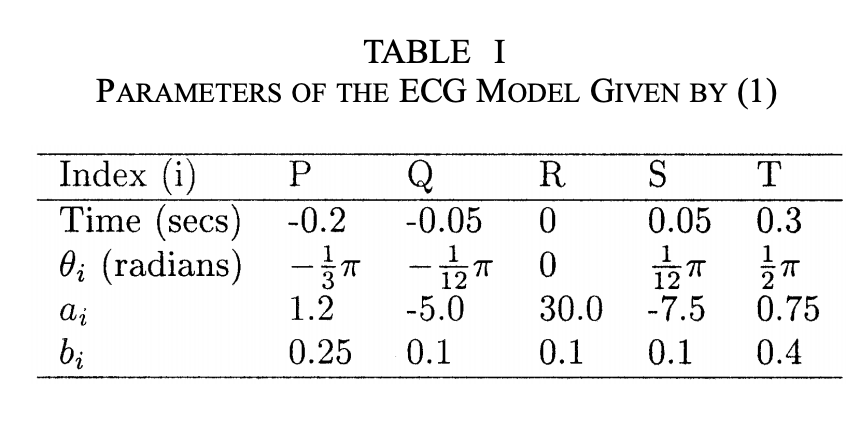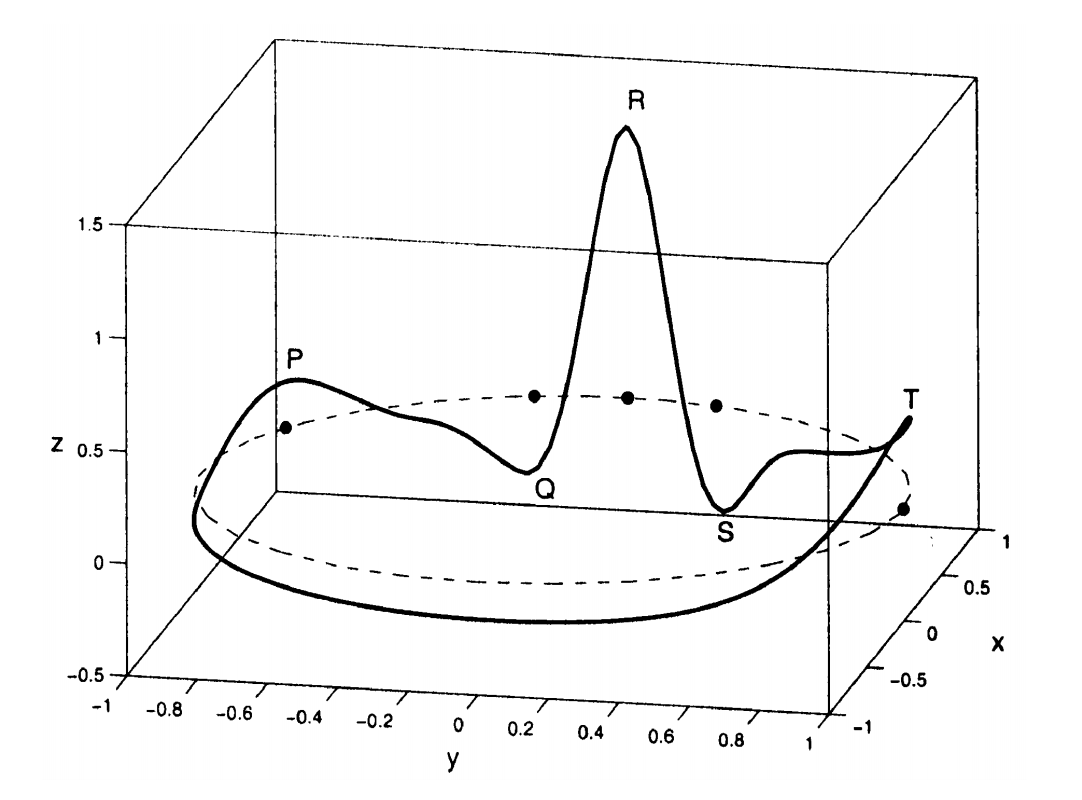This example can be referenced by citing the package.

import numpy as np
import neurokit2 as nk
import matplotlib.pyplot as plt

# This "decorative" cell should be hidden from the docs once this is implemented:
# https://github.com/microsoft/vscode-jupyter/issues/1182
plt.rcParams['figure.figsize'] = [15, 5]  # Bigger images
plt.rcParams['font.size']= 14


We can generate a normal multi-lead ECG signal with the following code:

ecg12 = nk.ecg_simulate(duration=10, method="multileads")

# Visualize results
ecg12[0:10000].plot(subplots=True);Abnormal ECG can be generated by modifying the simulation parameters. We will draw random parameters from a distribution.

# Normal parameters (used by default)
# ===================================
# t, the starting position along the circle of each interval in radius
ti = np.array((-70, -15, 0, 15, 100))
# a, the amplitude of each spike
ai = np.array((1.2, -5, 30, -7.5, 0.75))
# b, the width of each spike
bi = np.array((0.25, 0.1, 0.1, 0.1, 0.4))

# ===============
ti = np.random.normal(ti, np.ones(5) * 3)
ai = np.random.normal(ai, np.abs(ai / 5))
bi = np.random.normal(bi, np.abs(bi / 5))

ecg12 = nk.ecg_simulate(duration=10, method="multileads", ti=ti, ai=ai, bi=bi)

# Visualize results
ecg12[0:10000].plot(subplots=True);## Explanation#

For a better understanding of the above parameters, please read the following.We can see from the above table that each interval in the 3D trajectory can be fixed by 3 parameters:

• ti: The starting position $\theta_i/t_i$ along the circle of each interval in radius

• ai: The amplitude of each spike a

• bi: The width of each wave b. By altering these 3 parameters we can change the shape of the 3D trajectory and thus change the waveform of the resulting ECG.Prebuilt Synthetic Data Prebuilt synthetic data can be found at the following link”: